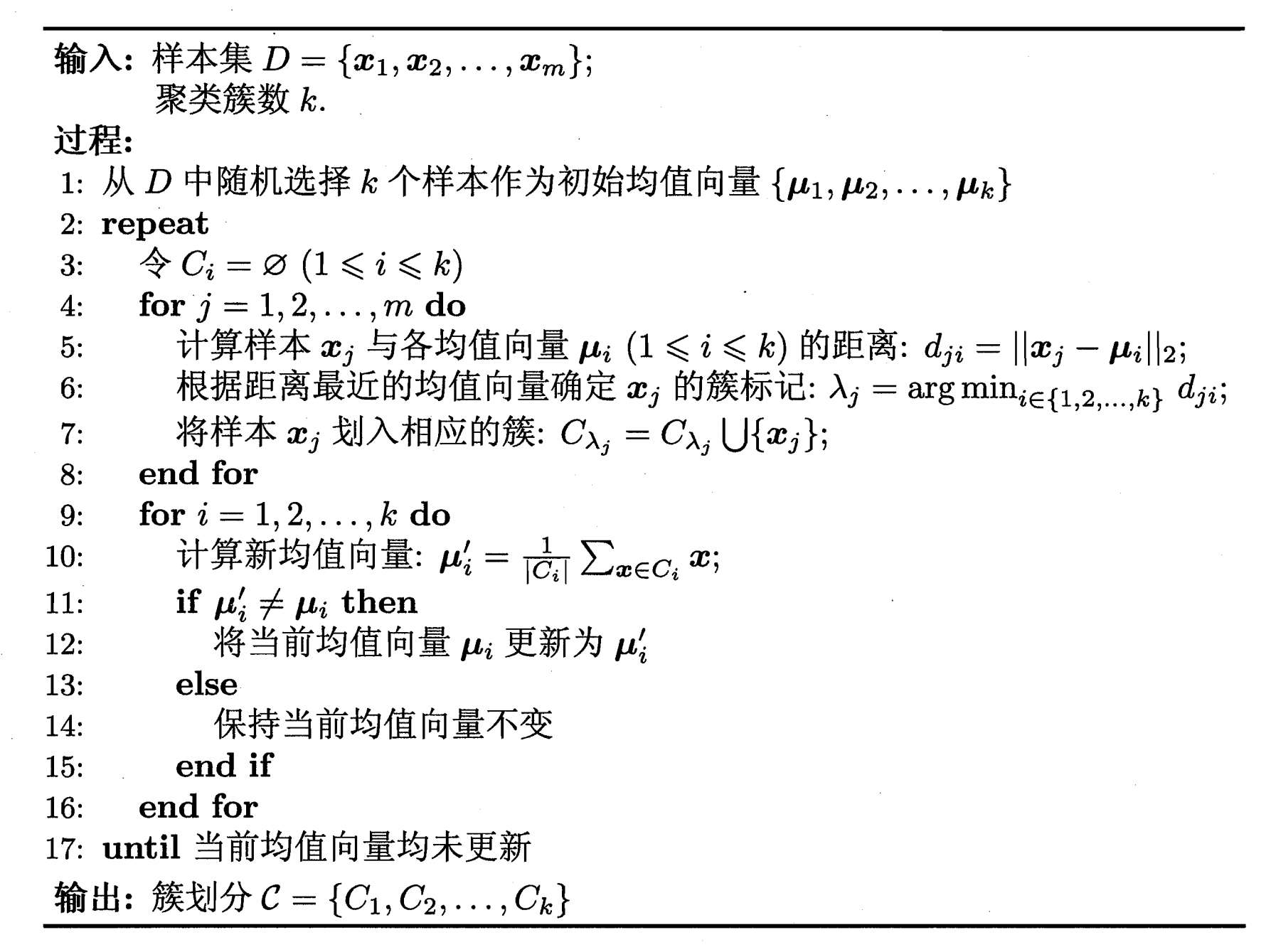## 性能度量

1. 将聚类结果和某个参考模型比较（例如某领域专家的划分），又称外部指标
2. 不考虑参考模型的内部指标

• Jaccard系数 $JC=\frac{a}{a+b+c}$
• FMI $FMI=\sqrt{\frac{a}{a+b} \times \frac{a}{a+c}}$
• Rand指数 $RI=\frac{2(a+d)}{m(m-1)}$
显然，这里指标都是越大越好。

## 距离计算

• 非负性$dist(x_i,x_j) >= 0$
• 同一性$dist(x_i,x_j)=0$ 当且仅当$i=j$
• 对称性$dist(x_i,x_j)=dist(x_j,x_i)$
• 直递性$dist(x_i,x_j)\le dist(x_i,x_k)+dist(x_k,x_j)$

## 聚类算法

### Kmeans

K-means算法针对给定样本集，对划分的簇最小化平方误差：k-means

Kmeans比较适合簇数已知的情况，且初始化簇中心会对算法结果造成很大影响，可以启发式地搜索k的取值，寻求拐点，但并不总是有效的。有很多种方法尝试更好的完成初始化，除了随机选择之外，还有按照经验选，按照密度选（计算每个样本一定空间内的样本数作为密度，选密度最大的作为第一个初始簇中心，离他一定距离之外选择此外最大密度作为第二个，以此类推）。